﻿ Application of Haar Wavelets and Method of Moments for Computing Performance Characteristics of Electromagnetic Materials

Application of Haar Wavelets and Method of Moments for Computing Performance Characteristics of Elec...

Vishwa Nath Maurya, Avadhesh Kumar MauryaOPEN ACCESSPEER-REVIEWED

Application of Haar Wavelets and Method of Moments for Computing Performance Characteristics of Electromagnetic Materials

Vishwa Nath Maurya1,, Avadhesh Kumar Maurya2

1Department of Mathematics, School of Science & Technology, University of Fiji, Fiji Ex-Professor & Director, Vision Institute of Technology, U.P. Technical University, India

2Department of Electronics & Communication Engineering Lucknow Institute of Technology, U.P. Technical University, India

Abstract

Present paper demonstrates on application aspect of Haar wavelets and method of moments for computing performance characteristics in electromagnetic field. In this paper, some significant results including performance characteristics relevant to application of the Haar wavelets as the expansion function in the method of moments have been successfully explored. The present analysis for performance evaluation has been focused for three different electromagnetic materials- finite straight, thin plane plate and eddy current problem. The main results explored are described in the main text and also concluded at the end with discussions.

At a glance: Figures

1234567
Prev Next

• Maurya, Vishwa Nath, and Avadhesh Kumar Maurya. "Application of Haar Wavelets and Method of Moments for Computing Performance Characteristics of Electromagnetic Materials." American Journal of Applied Mathematics and Statistics 2.3 (2014): 96-105.
• Maurya, V. N. , & Maurya, A. K. (2014). Application of Haar Wavelets and Method of Moments for Computing Performance Characteristics of Electromagnetic Materials. American Journal of Applied Mathematics and Statistics, 2(3), 96-105.
• Maurya, Vishwa Nath, and Avadhesh Kumar Maurya. "Application of Haar Wavelets and Method of Moments for Computing Performance Characteristics of Electromagnetic Materials." American Journal of Applied Mathematics and Statistics 2, no. 3 (2014): 96-105.

 Import into BibTeX Import into EndNote Import into RefMan Import into RefWorks

1. Introduction

Literature reveals that wavelets and different mathematical techniques have been applied in different frameworks rigorously by tremendous previous researchers. In the early of seventh decade, Harrington  studied time-harmonic electromagnetic fields and Phillips  presented numerical solution of certain integral equations occurring in electromagnetic. Later in 1965, Richmond  confined to explore digital computer solutions of the rigorous equations for scattering problems in electromagnetic. Subsequently keeping in view of increased interest of researchers in this direction Mittra  authored a noteworthy textbook on computer techniques for electromagnetic and Stoll  also succeeded to present a marvelous textbook on analysis of eddy currents. On the subject some other textbooks are available, for more details we refer Chui  and Constantine . It is noticeable that demand of applications of wavelets in electromagnetic engineering problems by previous researchers has been significantly increased since eighth decade and therefore a number of previous noteworthy researchers e.g. [3, 4, 5, 6, 8, 9, 12, 14, 19, 21, 28, 29, 34, 36, 37, 39, 42] confined their attention in this direction in order to analyze rigorously using different mathematical and computer techniques. Among them, Belardi et al. [3, 4] focused their keen attention on applications of Harrs wavelets for solving electrostatic problems and their computational aspects. Furthermore, it is universally accepted that different mathematical techniques have their wide applications in solving engineering problems including electromagnetic materials. Perhaps this may the reason why some earlier researchers e.g. Bossavit , Datta , Petre P. et al. [36, 37], Maurya [22-27] applied different mathematical techniques in different frameworks. To be more specific, Bossavit  used a numerical approach to transient nonlinear eddy current problems in applied electromagnetic field. Petre P. et al.  applied integral equation approach to analyze scattering from one-dimensional periodic coated strips. In this connection it is remarkable here that Harrington  succeeded to compute electromagnetic field using moment method.

Here, we are keenly interested to focus the main theoretical aspects of the method of moments and of the Haar wavelets for computing some significant performance characteristics in electromagnetic field.

2. The Method of Moments

As stated earlier that the method of moments is a mathematical technique having its numerical nature which has already been applied by some previous researchers e.g. Harrington , and hence the complete description and details of this method have already been presented in many papers, in order to guide the reader through the overall method explanation, a brief summary is here shown. In a simplified way, it can be mentioned that the basis of the method of moments is the application of approximation functions, like the following one, for more details we refer Harrington .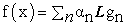(1)

In the aforementioned expression (1), αn is the unknown coefficients, gn is the expansion function, the pulse or the Haar wavelets, and “L” a mathematical operator. When the inner product is carried out using a weighed function Wm, then following expression can be found: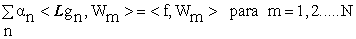(2)

The previous expression can be represented in a matrix form by [A][α][B], where [α] is the unknown approximated solution coefficients column vector, and the matrixes [A] and [B] are given by:(3)

As a first application, the potential distribution on a finite and straight wire that can be calculated using the next equation is taken into consideration in view of Clayton & Syed :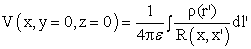(4)

Thus making use of the method of moments, knowing the approximated solution function f(x), the expansion function g(x) and the weighting function W(x), the potential on a finite straight wire can be estimated by the inner product of these functions:(5)

Consequently, the surface density can be approximated by the N term expansion. If the wire is divided into uniform segments Δ=L/N, after applying the weight delta function of Dirac Wm = δ( xm – x’) = 1, the inner product will become:(6)

Assuming the charges placed in the center of each subdivision in relation to the axis, substituting the values of x by the distance of the charge position to the point P(xm), we will have an integral that is only function of the x’. For a fixed potential V, the equation can be represented, using matrix notation, by [Vm] = [Zmn] [αn], in which Zmn is defined by Constantine :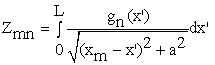(7)

The same approach can be used, if a two-dimensional application is considered. If a square plane plate is considered as an example, we should remember that the potential in a finite and very thin plane plate can be evaluated by Harrington :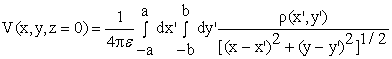(8)

Thus, after applying the method of the moments, knowing the function of the approximated solution f(x,y), the expansion function g(x,y) and the weighed function W(x,y), the potential in a square plane plate, will be estimated by the inner product of these functions Phillips :(9)

where,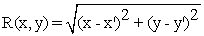(10)

Dividing the plate in equal segments and applying the weighed function as being the delta function of Dirac, we had that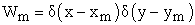, being the inner product in the point given by: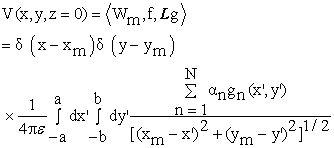(11)

Assuming the charges placed in the center of each sub division in relation to each axes, substituting the values of x and y by the distance of the charge position to the point P(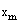,), we will have an integral that is only function of x' e. For a fixed potential V, the equation can be represented, using the matrix notation, by [Vm]=[Zmn][αn], in which Zmn is defined by:(12)

3. The Wavelets

The analysis through the wavelets has been a good alternative in replacement of the classical analyses that utilize the Fourier series, chiefly when treating acoustic signals, interpreting seismic signals and in the solution of numerical methods applied to electromagnetism and electrostatics. For further details we refer Cohen and Kovacevic , Liang et al. , Mallat . In general the wavelets can be defined by:(13)

Some kinds of wavelets are mentioned in the literature, making it possible for new family models to be built from them, which adapt more appropriately to each case. Figure 1 represents the Morlet or Modulated Gaussian wavelet, which is expressed by: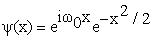(14)

The Figure 2 represents the Mexican hat wavelet, which is expressed by: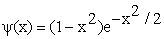(15)

The Figure 3 represents the Shannon wavelet, which is expressed by:(16)

4. The Haar Wavelets

It was previously mentioned that many functions can be used as the expansion function: Among them, the pulse function, the truncate cosine function and the wavelets can be mentioned. Thus, after applying the method of moments, and considering the Haar wavelets, a function f(x,y) can be approximated by: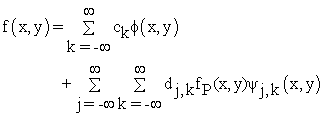(17)

In this equation “j”, and “k” are the resolution and the translation levels, respectively.

Moreover, once the Haar wavelets, and the so-called mother function and scale function father are applied, the formulation, for two-dimensional applications, will result in a product combination, given by Morettin [32, 33]:(18)(19)

5. Mathematical Formulation

5.1. Case I: Finite Straight

Thus, making use of the method of moments, and the wavelets the Haar the potential on a finite straight wire can be estimated by the inner product of these functions. As an illustration, the Figure 4 represents the Haar function regarding one dimensions and two level of resolution, for more details we Newland .

The mathematical solution is: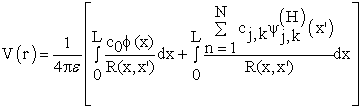(20)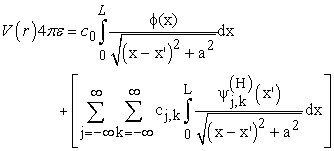(21)
5.2. Case II: Thin Plane Plate

The same approach can be used, if a two-dimensional application is considered.

It was previously mentioned that many functions can be used as the expansion function. Among them, the pulse function, the truncate cosine function and the wavelets, the general aspects of the wavelets are shown.

As an illustration, the Figure 5 represents the Haar function regarding two dimensions and one level of resolution, for a point P(xm, ym).

On the other hand, if the potential in a finite and very thin plane plate is taken into account as an application, it can be evaluated in the light of Aboufadel and Schlicker :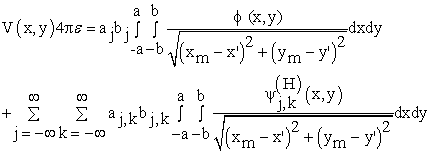(22)

At each point we have:(23)
5.3. Case III: Eddy Current Problem

Let us consider the conducting wire to be composed of filaments, having their length is represented by c and current density by J(r’), according to Figure 6.

The two-dimensional fields can be obtained by using two or more current distributions J1, J2... on surface S, which is the interface between the conductor and the air.

By integrating it is possible to simplify the equations the most, so that there will not be complex integrals or approximations, as follows Kriezis et al.  and Lopez :(24)

In which r and r´ are respectively the origin and source points. The current density can be expressed by: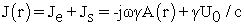(25)

In which U0 is the applied voltage, c and s are the length and the sectional area of the conductor. Considering Fredholm integral, a second order equation is obtained:(26)

In which the math operator in the previous equation is given by: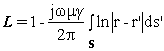(27)

By dividing the domain into N elements, the current density can be approximated by: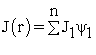(28)

By choosing the pulse as the expansion and weighting functions, the coefficients of matrix A can be determined by the following expression in view of Kaitec  and Stoll :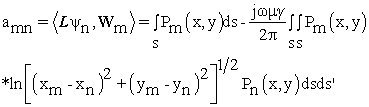(29)

or alternatively we have as following: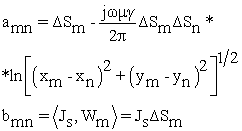(30)

Let us consider the sides of the elements resulting of the divisions performed in the superficial part of the conductor are square and defined as h.

By eliminating the other ΔSm terms in the equations amn and bm, considering the relative positions of the different charges that will form the elements of matrix A, and the relations d/h> 2 and d/h=1, the following will be obtained:(31)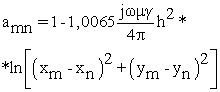(32)

if m = n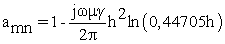(33)

By solving matrix A{J}={Js} the current matrix will be obtained.

6. Application and Observational Discussions

6.1. Case I: Finite Wire

Applying the aforementioned formulation, we got some results related to two applications: the first one related to a finite and straight wire, and another one regarding a thin plane plate. It is assumed in the two applications a constant potential distribution equal to1V, conform Figure 7.

Table 1 presents the results regarding the charge surface density on a 1.0m straight wire, when it is divided in to 16 equal segments, as a function of the resolution (j) and the translation (k) levels. It is remarkable here that Belardi et al.  suggested in their rigorous study that these results can be considered as the ones suitable to validate this approach.

Table 1. Charge surface density (pc/m) on straight finite as a function of the resolutions levels

6.2. Case II: The Thin Plane Plate

After applying the aforementioned formulation, some results were obtained. For example, the Figure 8 represents the surface charge density in a square plate (1.0mx1.0m), submitted to a potential of 1.0 V. In this case, it was adopted 16 subdivisions for each of the axes, and the level 5 of resolution was applied to the Wavelets. Concerning the characteristic of the method, it should be emphasized that the application of the Haar wavelets originates scattered matrixes. Thus, we will have nulls coefficients that can result in a computing time reduction.

The Table 2 depicts the comparative results, regarding the computing time values function of the adopted axe division number, with or without applying the null value detection routine as studied by Belardi .

Table 2. Computing time(s) function of the axe subdivisions and of the null value detection use

In applying for a finite flat plate, we measured the execution time of the program, varying the number of divisions in each of the axes, measuring both the amount held in floating point operations as the runtime. The Table 3 illustrates the values obtained for the total execution time and the amount of floating point operations performed, using as expansion function the Haar wavelet.

Table 3. Calculation floating point operations and the execution time depending on the number of divisions of the plate

Taking advantage of the fact that the Haar matrix is sparse, we reduce the execution time of the program by entering a comparison that, when the null value is detected, the transaction between the arrays is performed. The Table 4 presents the results comparing the values of the runtime and the number of floating point operations, with and without detection of null values.

Table 4. Amount of floating point operations and execution time (s), depending on the number of divisions of the plate with and without detection of nulls

According to the results reduced on average 40% run time of the program Figure 9 (blue color) shows the values obtained for the runtime with and without detection of null values, depending on the number of divisions of finite flat plate.

When the plate was divided into 16 equal segments on each axis, a total of 256 coefficients were generated with 54% of them are zero. Taking advantage of the fact that the Haar matrix is sparse, applying the matrix algebra we can write that(34)

where, Zmn is a square matrix that is not necessarily a scattered one, since it depends on the expansion function that was chosen. Thus, taking advantages of the fact that the Haar matrix is a scattered matrix, applying the matrix algebra, it will result e.g. Datta :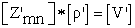(35)

else,(36)(37)(38)

As estimation, when the null value detection routine is carried using the null value detection routine. The Figure 10 represents the Haar matrix and Figure 11 and Figure 12 presents the Zmn matrix configuration for the threshold equal to 0.01%, and 0.05%, respectively. The dark part is the no null values.

Table 5. Computing time(s) as a function of the axe subdivisions and of the adopted threshold level

The Figure 13 represents the error variation for the charge surface density, considering a square plane plate, and 16 axe subdivisions, as a function of the selected threshold.

Therefore, the variation of the threshold allowed a significant reduction in execution time without significantly changing the value of the surface charge density.

Moreover, it should be mentioned that the Cholesky decomposition method were also implemented as referred in Cohen and Kovacevic . The Figure 14 represents the matrix configuration after applying it, assuming a threshold level equal to 0.01%. In this case, approximate increase of 64% was obtained in the null value element of the matrix.

Regarding the computational performance, the average computing time decreased from 0.21 to 0.02 (s), for 16 axe subdivision, and a reduction time from 11.49 to 0.351(s).

6.3. Case III: Eddy Current Problem

In the application here presented, a copper conductor with the conductivity of 1.72 (μΩcm) and resistivity of 100% as shown on Table 6, we refer KPS .

Table 6. Material Characteristics

The Figure 15 shows the reactions of the electromagnetic field and the involved energy considering the influence between the charges using the developed program.

Therefore the final equation for each one of the elements that compose the current matrix can be expressed by:(39)

The solution of the previous equation can be obtained by using the expression [amn]*[Coef]=[U0], we refer Bossavit  and Salon and Peng  for more details in this connection.

The Figure 16 shows the superficial charge distribution on the conductor, taking into consideration the effects of losses.

The Figure 17 was obtained through the use of the application toolbox and shows the results of the coefficients in relation to the several resolution levels. The numerical values are available in the application as well as explored by Palm .

The Figure 18 shows the statistical data for a 0.01% threshold with level 5 resolution.

Figure 19. Superficial charge distribution with different resolution levels

The Figure 19 shows several the coefficients in different resolution levels from matrix amn.

7. Discussions and Conclusions

Present research article qualifies to demonstrate successfully some significant performance characteristics of for three different electromagnetic materials-finite straight, thin plane plate and eddy current problem by using Harr wavelets and method of moments. A series of applications of wavelets, such as the surface density in a finite straight wire and a flat plate, and the determination of eddy currents using the pulse and as a expansion function of the Haar wavelet have been illustrated by plotting graphs.The proposed methodology herein enables to search out the available numerical coefficients in the application as a function of the resolution level, this way avoiding the complex solution of the inner product, usually composed of double integrals that do not possess a very immediate solution.

Furthermore, performing the product of the current matrix by the Haar wavelet [amn][Coefficients][Haar] = [U0][Haar] and in some cases reductions in execution time of up to 40% has been achieved. With this reduction in run time no significant variation in the amn elements that could compromise the final results has been found.

Although the proposed application is relatively simple, the presented methodology is likely to be applied to problems of greater complexity, such as a refinement can be achieved in energy in locking electric motors, cardiac signals, transmission lines, electromagnetic compatibility, financial market, corrosion or thermal treatment, neurological treatments and etc.

References

  Aboufadel E. and Schlicker S., Discovering Wavelets, John Wiley & Sons, pp. 1-42, 1999.In article CrossRef  Balanis C.A., Antenna Theory: Analysis and Design, Harper & Row, New York, pp. 283-321, 1982.In article  Belardi A.A., Cardoso J.R., and Sartori C.F., Application of Haar’s Wavelets in the method to solve electrostatic problems, Compel Vol. 23(3), pp. 606-612, 2004In article CrossRef  Belardi A.A., Cardoso J.R., and Sartori C.F., Wavelets Application in Electrostatic and their Computing Aspects, Electric and Magnetic Fields, EMF, Germany, pp. 43-46, 2003In article  Bossavit A., A numerical approach to transient nonlinear eddy current problems in applied electromagnetic in materials, Elsevier, 1990.In article  Bulter C.M. and Wilton D.R., Analysis of various numerical techniques applied to thin-wire scatterers, IEEE Trans,Vol. AP-23 (4), pp. 524-540, July 1975.In article  Chui C.K., An Introduction to Wavelets, Academic, New York, 1991.In article  Clayton P.R. and Syed N. A., Introduction to Electromagnetic Fields, 1st Edition, New York, McGraw-Hill, 1998.In article  Cohen A. and Kovacevic J., Wavelets: The mathematical background, IEEE Proceedings of the IEEE, Vol. 84 (4), pp.514-522, 1996.In article  Constantine A.B., Advanced Engineering Electromagnetics, 2nd Edition, New York, John Wiley & Sons, 1989.In article  Datta B.N., Numerical Linear Algebra and Applications, 1st Edition, New York, Brooks/Cole Publishing Company, pp. 222-225, 1995In article  Daubechies I., Ten Lectures on Wavelet, SIAM Press, Philadelphia, 1992.In article CrossRef  Harrington R.F., Field Computation by Moment Method, Macmillan Press, New York, 1968.In article  Harrington R.F., Time-Harmonic Electromagnetic Fields, Graw-Hill, New York, 1961.In article  Kaitec, The 14th Kori Unit 4 Steam Generator Tube Eddy Current Examination – Final Report, Nov., 2004.In article  Kishk A.A., Glisson A.W. and Goggans P.M., Scattering from conductors coated with materials of arbitrary thickness, IEEE Trans. Antennas Propagat., Vol. AP-40, pp. 108-112, Jan. 1992.In article CrossRef  KPS, The 5th Urchin Unit 4 Steam Generator Tube Eddy Current Examination – Final Report, Mar. 2005.In article  Kriezis E.E., Stavaros M.P., and Tegopoulos J.A., Eddy Currents: Theory and Applications, Proceedings of the IEEE, Vol. 80(10), 1992.In article CrossRef  Liang J., Elangovan S., Devotta J.B.X., A wavelet multiresolution analysis approach to fault detection and classification in transmission lines. Elsevier Computer Physics Communications, pp.327-332, 1999.In article  Lopez L.A. N. M., Transformadas de Wavelet e Lógica Fuzzy na Inspeção por Eddy-Current em Tubos de Geradores de Vapor de Centrais Nucleares, Tese de Doutorado,Universidade de São Paulo, USP, Brasil, 2003.In article  Mallat S., Wavelets for a vision. IEEE Proceedings of the IEEE, Vol. 84(4), pp.604-614, 1996.In article  Maurya A.K., Maurya V.N. & Singh R.K., Computational approach for performance analysis of photonic band gap structure on defected ground surface with microwave and band stop filter, American Journal of Engineering Technology, Academic & Scientific Publishing, New York, USA, Vol. 1, No. 7, pp. 10-18, 2013.In article  Maurya Avadhesh Kumar & Maurya V.N., A novel algorithm for optimum balancing energy consumption LEACH protocol using numerical simulation technique, International Journal of Electronics Communication and Electrical Engineering, Algeria, Vol. 3, Issue 4, pp. 1-19, 2013 a.In article  Maurya Avadhesh Kumar & Maurya V.N., Linear regression and coverage rate analysis for optimization of received signal strength in antenna beam tilt cellular mobile environment, International Journal of Electronics Communication and Electrical Engineering, Algeria, Vol. 3, Issue 7, pp. 1-14, 2013 b.In article  Maurya Avadhesh Kumar & Maurya V.N., The teleronci model on elementary particles of atomic nucleus- an experimental approach, American Journal of Engineering Technology, Academic & Scientific Publishing, New York, USA, Vol.1, No. 9, December 2013 c, pp. 19-27.In article  Maurya Avadhesh Kumar, Maurya Vishwa Nath, Singh R.K., A novel method for analysis of synchronization of GPS and geosynchronous satellite signals using solar braking and intrinsic velocity rectification, Journal of Engineering and Technology Research, Scientia Research Library, Georgia, Vol. 2, Issue 1, pp. 17-24, 2014.In article  Maurya V.N., Numerical simulation for nutrients propagation and microbial growth using finite difference approximation technique, International Journal of Mathematical Modeling and Applied Computing, Academic & Scientific Publishing, New York, USA, Vol. 1, No.7, November 2013, pp. 64-76.In article  Medgyesi-Mitschang L.N. and Putnam J.M., Electromagnetic scattering from electrically large coated flat and curved strips: Entire domain Galerkin formulation, IEEE Trans. Antennas Propagat., Vol. AP-35, pp.790-801, July 1987.In article CrossRef  Medgyesi-Mitschang L.N. and Wang D.S., Hybrid solutions for scattering from large bodies of revolution with material discontinuities and coatings, IEEE Trans. Antennas Propagat., Vol. AP-32, pp. 717-723, June 1984.In article CrossRef  Meyer Y., Wavelets: Algorithms & Applications, translated and revised by R. D. Ryan, SIAM Press, Philadelphia, 1993.In article  Mittra R., Computer Techniques for Electromagnetics, Pergamon Press, Oxford, 1973.In article  Morettin P.A., 7 Escola de Séries Temporais e Econometria, 1st Edition, São Paulo, Edusp, pp. 20-34, 1997.In article  Morettin P.A., Ondas e Ondaletas 1°ed, São Paulo, Edusp, pp. 1-55, 1999.In article  Newland D.E., Random Vibrations Spectral and Wavelet Analysis, Addison Wesley Longman, pp. 315-333, 1993.In article  Palm W., Introduction to Matlab 7 and Simulink for Engineers, McGraw Hill, pp. 55-97, 2003.In article  Petre P., Swaminathan M., Veszely G. and Sarkar T.K., Integral equation solution for analyzing scattering from one-dimensional periodic coated strips, IEEE Trans. Antennas Propagat., Vol. AP–41, pp.1069-1080, Aug. 1993.In article CrossRef  Petre P., Swaminathan M., Zombory L., Sarkar T.K. and Jose K.A., Volume/surface formulation for analyzing scattering from coated periodic strip, IEEE Trans. Antennas Propagat., Vol. AP–42, pp. 119-122, Jan. 1994.In article CrossRef  Phillips B.L., A technique for the numerical solution of certain integral equations of the second kind, 1962.In article  Rao S.M., Cha C.C., Cravey R.L. and Wilkes D.L., Electromagnetic scattering from arbitrary shaped conducting bodies coated with lossy materials of arbitrary thickness, IEEE Trans. Antennas Propagat., Vol. AP-39, pp. 627-631, May 1991.In article CrossRef  Richmond J.H., Digital computer solutions of the rigorous equations for scattering problems, Proc. IEEEE, Vol. 53, pp.796–804, Aug.1965.In article  Salon S. and Peng J.P., Three dimensional eddy currents using a four component finite element formulation, IEEE Transactions on Magnetics, 1994.In article  Shu C. and Sarkar T.K., Electromagnetic scattering from coated strips utilizing the adaptive multi scale moment method, Electrical Engineering and Computer Science, pp. 173-208, 1998.In article  Stoll R.L., The analysis of eddy currents, Clarendon Press, 1974.In article